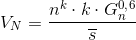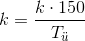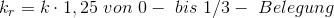## Nominal flow volume

Filling a mold cavity within a certain time requires a particular flow volume in cm³/s. Therefore, Friedrich Nielsen turned to calculating the so-called nominal flow volume VN. He determined this parameter from the casting weight GN in kg, the mean wall thickness s in cm, the number of mold cavities n and an additional process- and casting temperature-dependent factor k.The nominal volume flow is calculated as follows according to equation 1 below:

Eq. 1:GN = raw casting weight in kg
s = mean wall thickness in cm

The k-values in Table 1 apply to casting temperatures of 150°C above the liquidus temperature of the cast melt. If the intended superheat is greater or less than 150°C, the factor needs to be adjusted according to eq. 2:

Eq. 2:Ts = melt superheat in K

Moreover, for aluminum gravity die casting (see Gravity die casting process), a k-value of 160 only applies at a surface temperature of 330 to 350°C prior to casting.

If a mold is provided with internal chills for aluminumsand casting, the cooling rate increases. Accordingly, Nielsen proposes doubling of the k-value for molds fully occupied with internal chills. He considers partial occupation with internal chills as follows (eq. 3):

Eq. 3:k2r = kr*1.25 from 1/3 to 2/3 occupation
k3r = kr*1.28 from 2/3 to full occupation